# Sample Data: Waterstriders 3

Locations of waterstrider larvae

## Details

Locations of waterstrider larvae in the observation region Rectangle[{0., 0.}, {46.4, 46.4}] centimeters without annotations.

## Examples

### Basic Examples (2)

Retrieve the data:

 In:=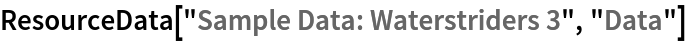Out=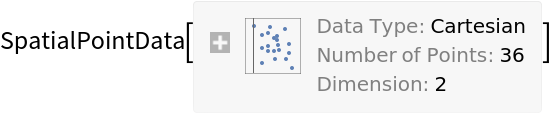Summary of the spatial point data:

 In:=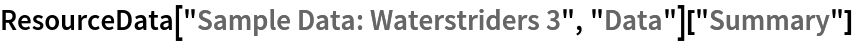Out=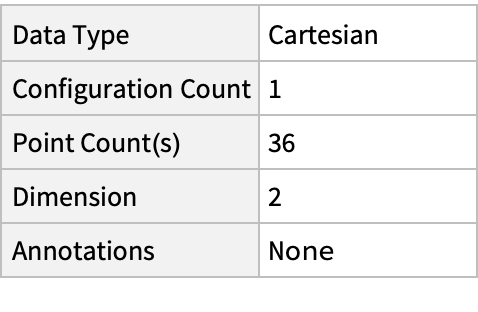### Visualizations (2)

Plot the spatial point data:

 In:=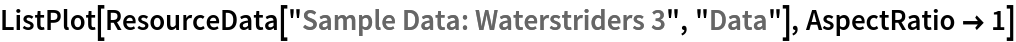Out=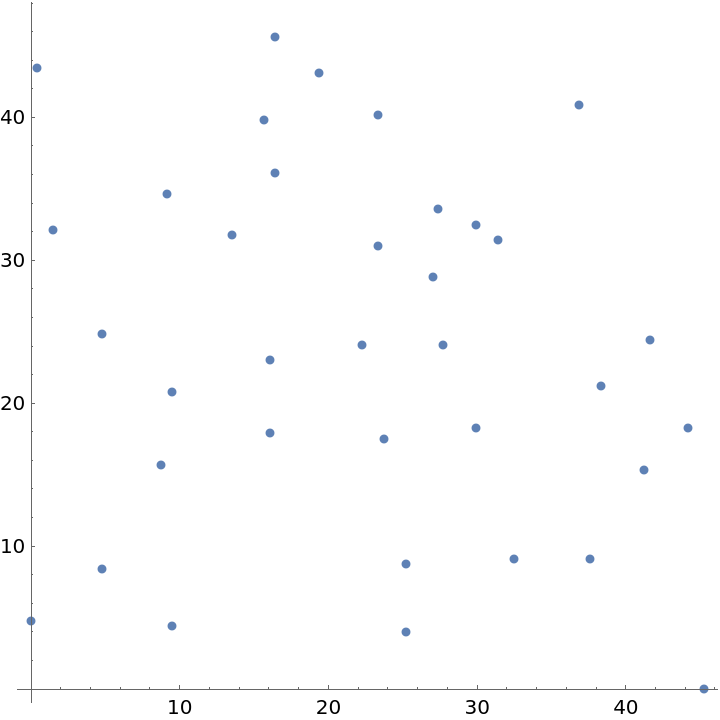Visualize the smooth point density:

 In:=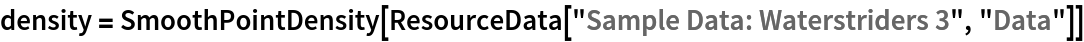Out=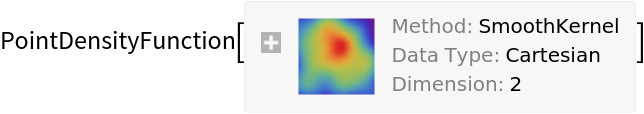In:=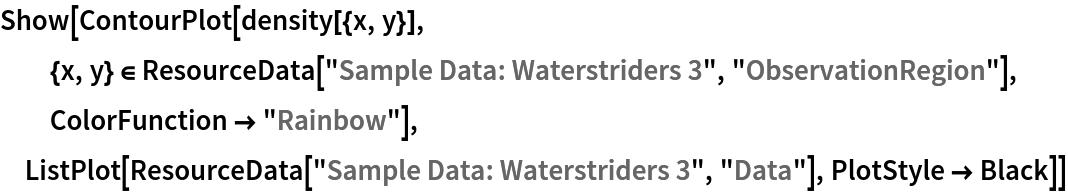Out=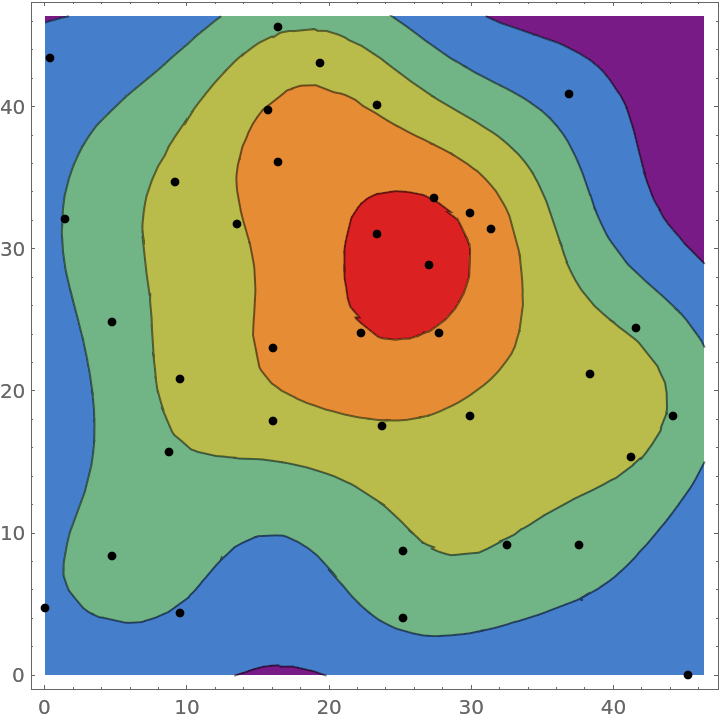### Analysis (4)

Compute probability of finding a point within given radius of an existing point - NearestNeighborG is the CDF of the nearest neighbor distribution:

 In:=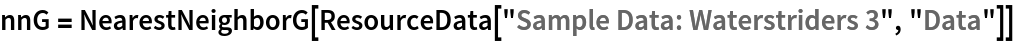Out=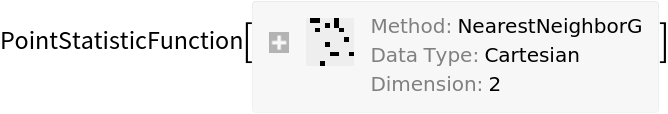In:=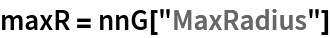Out=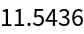In:=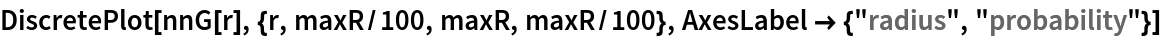Out=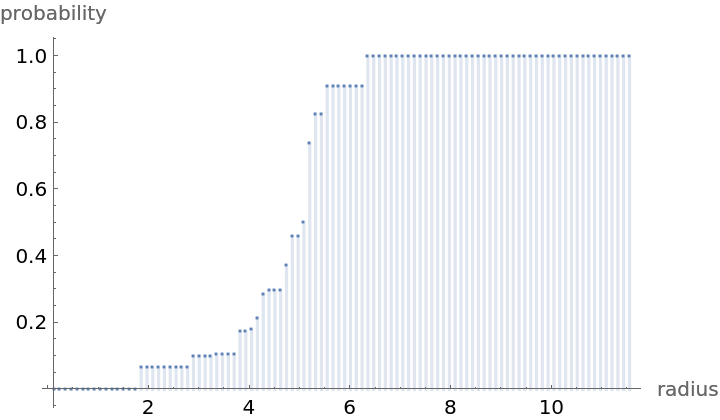Mean distance between a typical point and its nearest neighbor (for positive support distribution can be approximated via a Riemann sum of 1-CDF):

 In:=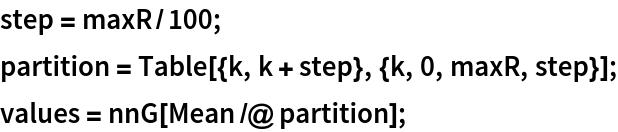In:=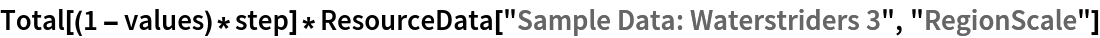Out=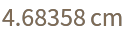Test for complete spatial randomness:

 In:=Out=Fit a Poisson point process to data:

 In:=Out=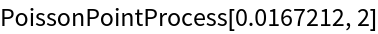Gosia Konwerska, "Sample Data: Waterstriders 3" from the Wolfram Data Repository (2021)